• 如何用typedef定义结构体数组
千次阅读 多人点赞
2018-07-05 10:54:39

今天在看图的邻接表时，看到了这样一段代码

typedef struct VertexNode
{
char data;
int weitht;
struct EdgeNode * firstEdge;
}VertexNode,AdjList[MAX_VERTEX];

再比如

typedef int arr;

arr a;就定义了一个有5个int型变量的数组a。

更多相关内容
• C语言定义结构体数组 typedef struct vnode // 顶点结构 { VertexType vex; // 存储顶点名 EdgeNode *firstedge; // 边表头指针，指向顶点第一个邻接点 } VertexNode, AdjList[MAX_VERTEX_NUM]; typedef struct ...

# C语言定义结构体数组

typedef struct vnode // 顶点结构
{
VertexType vex;      // 存储顶点名
EdgeNode *firstedge; // 边表头指针，指向顶点第一个邻接点

typedef struct {
int vexnum;      // 节点的数目
int edgenum;     // 边的数目
} ALGraph;
————————————————
版权声明：本文为CSDN博主「其铄」的原创文章，遵循CC 4.0 BY-SA版权协议，转载请附上原文出处链接及本声明。
原文链接：https://blog.csdn.net/weixin_42250302/article/details/89500638


## typedef 关键字的用法

typedef int array[MaxSize];
typedef array* prear;
typedef prear array10;
array togs;//togs是具有五个int 类型元素的数组
prear p2;//表示p2是一个指针，指向一个array类型的数组
prear ap;//表示ap是存放有10个指向array类型的指针的指针数组//ap是具有是个指针元素的指针数组\每个指针作为指针数组的元素指向具有5个int类型元素的array数组


**所以typedef struct vnode AdjList[MAX_VERTEX_NUM]**表示：定义一个具有MAX_VERTEX_NUM个vnode类型的元素的数组；

AdjList adjlist;


展开全文数据结构 学习 b树
• （1）结构体定义使用 基本概念：结构体属于用户自定义的数据类型，允许用户存储不同的数据类型。 参考视频：https://www.bilibili.com/video/BV1et411b73Z?from=search&seid=4205594350351753444 已投币三...

# 十二、结构体

（1）结构体定义和使用

基本概念：结构体属于用户自定义的数据类型，允许用户存储不同的数据类型。

已投币三连，非常好的视频教程，感谢up主的奉献。

结构体定义和使用：

语法：

struct  结构体名  { 结构体成员列表 };

通过结构体创建变量的方式有三种：

struct  结构体名  变量名;
struct  结构体名  变量名 = { 成员1值, 成员2值... };
定义结构体时顺便创建变量;

代码：

/* ------------------ 1.struct  结构体名  变量名; 之后进行赋值操作 ------------------ */
struct Student
{
// 成员列表
string name;    // 姓名
int age;	// 年龄
int score;	// 分数
};

int main() {

//struct Student xiaoMing;
Student xiaoMing;	// struct关键字可以省略

xiaoMing.name = "小明";
xiaoMing.age = 18;
xiaoMing.score = 96;

xiaoMing = { "小明", 18, 96 };

cout << "姓名：" << xiaoMing.name << "  年龄：" << xiaoMing.age << "  分数：" << xiaoMing.score << endl;

system("pause");
return 0;
}

/* -------------- 2.struct  结构体名  变量名 = { 成员1值, 成员2值... }; -------------- */
struct Student
{
// 成员列表
string name;    // 姓名
int age;	// 年龄
int score;	// 分数
};

int main() {

Student xiaoMing = {"小明", 18, 96};

cout << "姓名：" << xiaoMing.name << "  年龄：" << xiaoMing.age << "  分数：" << xiaoMing.score << endl;

system("pause");
return 0;
}

/* -------------------- 3.定义结构体时顺便创建变量; -------------------- */
struct Student
{
// 成员列表
string name;    // 姓名
int age;	// 年龄
int score;	// 分数
};

int main() {

xiaoMing.name = "小明";
xiaoMing.age = 18;
xiaoMing.score = 96;

xiaoMing = { "小明", 18, 96 };

cout << "姓名：" << xiaoMing.name << "  年龄：" << xiaoMing.age << "  分数：" << xiaoMing.score << endl;

system("pause");
return 0;
}
-----------------------------------------------------------------------------------
姓名：小明  年龄：18  分数：96
请按任意键继续. . .



使用 typedef 定义 struct 结构体方法https://blog.csdn.net/Mark_md/article/details/107656790

（2）结构体数组

作用：将自定义的结构体放入到数组中，方便维护。

语法：

struct  结构体名  数组名[ 元素个数 ] = { {}, {}, ... {} };
struct Student
{
// 成员列表
string name;    // 姓名
int age;	// 年龄
int score;	// 分数
};

int main() {

struct Student stuArray =
{
{ "小明", 18, 96 },
{ "小红", 20, 100 },
{ "小亮", 21, 98 }
};

for (int i = 0; i < 3; i++)
{
cout << "姓名：" << stuArray[i].name
<< "  年龄：" << stuArray[i].age
<< "  分数：" << stuArray[i].score << endl;
}

system("pause");
return 0;
}
----------------------------------------------------------------------------
姓名：小明  年龄：18  分数：96
姓名：小红  年龄：20  分数：100
姓名：小亮  年龄：21  分数：98
请按任意键继续. . .

（3）结构体指针

作用：通过指针访问结构体中的成员。

利用操作符 -> 可以通过结构体指针访问结构体属性。

示例：

struct Student
{
// 成员列表
string name;    // 姓名
int age;	// 年龄
int score;	// 分数
};

int main() {

struct Student xiaoMing = { "小明", 18, 96 };
Student* p = &xiaoMing;

cout << "姓名：" << p->name
<< "  年龄：" << p->age
<< "  分数：" << p->score << endl;

system("pause");
return 0;
}
-----------------------------------------------------------------
姓名：小明  年龄：18  分数：96
请按任意键继续. . .

（4）结构体嵌套

结构体嵌套结构体：

作用：结构体中的成员可以是另一个结构体。

例如：每个老师辅导一个学员，老师的结构体中，记录学生的结构体。

示例：

struct student
{
// 成员列表
string name;    // 姓名
int age;	// 年龄
int score;	// 分数
};

struct teacher
{
int id;			// 职工编号
string name;		// 教师姓名
int age;		// 教师年龄
struct student stu;	// 子结构体 学生
};

int main() {

teacher wang;

wang.id = 112374;
wang.name = "王芳";
wang.age = 26;
wang.stu.name = "小明";
wang.stu.age = 18;
wang.stu.score = 96;

cout << "教师姓名：" << wang.name
<< "  教师年龄：" << wang.age
<< "  教师ID号：" << wang.id << endl;
cout << "学生姓名：" << wang.stu.name
<< "  学生年龄：" << wang.stu.age
<< "  学生分数：" << wang.stu.score << endl;

system("pause");
return 0;
}
-------------------------------------------------------------------------
教师姓名：王芳  教师年龄：26  教师ID号：112374
学生姓名：小明  学生年龄：18  学生分数：96
请按任意键继续. . .

（5）结构体做函数参数

作用：将结构体作为参数向函数中传递

传递方式分两种：值传递，地址传递。

值传递示例：

struct student
{
// 成员列表
string name;    // 姓名
int age;	// 年龄
int score;	// 分数
};

void printStudent(student stu)
{
cout << "姓名：" << stu.name
<< "  年龄：" << stu.age
<< "  分数：" << stu.score << endl;

stu.name = "无";
stu.age = 0;
stu.score = 0;
}

int main() {

student xiaoMing = { "小明", 18, 96 };

printStudent(xiaoMing);

cout << "姓名：" << xiaoMing.name
<< "  年龄：" << xiaoMing.age
<< "  分数：" << xiaoMing.score << endl;

system("pause");
return 0;
}
---------------------------------------------------------------------------
姓名：小明  年龄：18  分数：96
姓名：小明  年龄：18  分数：96
请按任意键继续. . .

地址传递示例：

struct student
{
// 成员列表
string name;    // 姓名
int age;	// 年龄
int score;	// 分数
};

void printStudent(student * stu)
{
cout << "姓名：" << stu->name
<< "  年龄：" << stu->age
<< "  分数：" << stu->score << endl;

stu->name = "无";
stu->age = 0;
stu->score = 0;
}

int main() {

student xiaoMing = { "小明", 18, 96 };

printStudent(&xiaoMing);

cout << "姓名：" << xiaoMing.name
<< "  年龄：" << xiaoMing.age
<< "  分数：" << xiaoMing.score << endl;

system("pause");
return 0;
}
--------------------------------------------------------------------------
姓名：小明  年龄：18  分数：96
姓名：无  年龄：0  分数：0
请按任意键继续. . .

如果不想实参结构体内容，用值传递，反之用地址传递。

地址传递还可以使用数组，可实现同样功能，书写与阅读比指针更加直观。

（6）结构体中const使用场景

作用：用const来防止误操作

值传递会copy，会占内存空间，复制也会多花时间。所以如果可以用地址传递，可以提高执行效率。

而地址传递可能会对原始对象进行误修改，这时候就可以加 const。如果出现修改，编译器会报错

示例：

void printStudent(const student * stu)
{
cout << "姓名：" << stu->name
<< "  年龄：" << stu->age
<< "  分数：" << stu->score << endl;

stu->name = "无";    // 编译器会报错
stu->age = 0;
stu->score = 0;
}

展开全文c++
• 结构体数组 结构体数组格式：声明变量类型 数组名称[数组长度] //定义一个结构体数组名为a,它里面包含有5个元素，每一个元素都是一个结构体变量 Wor a={}; ...

# 结构体数组

#### 结构体数组的定义：

结构体数组格式：声明变量类型 数组名称[数组长度]

//定义一个结构体数组名为a,它里面包含有5个元素，每一个元素都是一个结构体变量
Wor a={};

#include<stdio.h>
typedef struct worker{
char id;
char name;
char sex;
int pay;
}WOR;
void main(){
WOR a=
{
{"0001","陈文杰","男",1200},
{"0001","张三丰","女",500},
{"0001","张四丰","女",1200}
}
}


对比记忆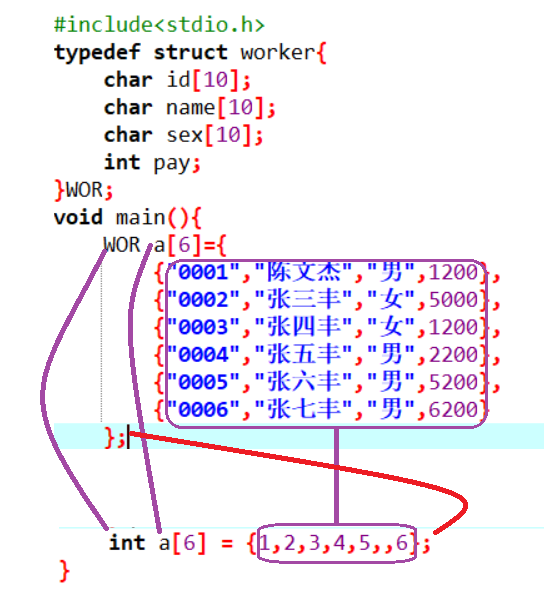#### 结构体数组的输出

#include<stdio.h>
typedef struct worker{
char id;
char name;
char sex;
int pay;
}WOR;
void main(){
WOR a={
{"0001","陈文杰","男",1200},
{"0002","张三丰","女",5000},
{"0003","张四丰","女",1200},
{"0004","张五丰","男",2200},
{"0005","张六丰","男",5200},
{"0006","张七丰","男",6200}
};
int i;
for(i=0;i<6;i++){
printf("%s,%s,%s,%d\n",a[i].id,a[i].name,a[i].sex,a[i].pay);
}
}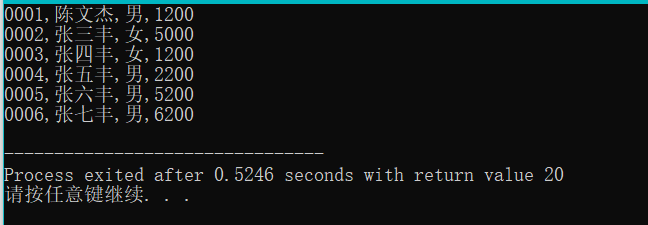结构体对象中的a[i]就代表一个对象，它已经不再是一个值了
所以我们应该用a[ii].成员名

改进以上程序

#include<stdio.h>
typedef struct worker{
char id;
char name;
char sex;
int pay;
}WOR;
void output(WOR a[],int n){//次函数是用来输出长度为n的工人数组的信息
int i;
printf("工号\t姓名\t性别\t年龄\n");
for(i=0;i<6;i++){
printf("%s\t%s\t%s\t%d\n",a[i].id,a[i].name,a[i].sex,a[i].pay);
}
}
void main(){
WOR a={
{"0001","陈文杰","男",1200},
{"0002","张三丰","女",5000},
{"0003","张四丰","女",1200},
{"0004","张五丰","男",2200},
{"0005","张六丰","男",5200},
{"0006","张七丰","男",6200}
};
output(a,6);
}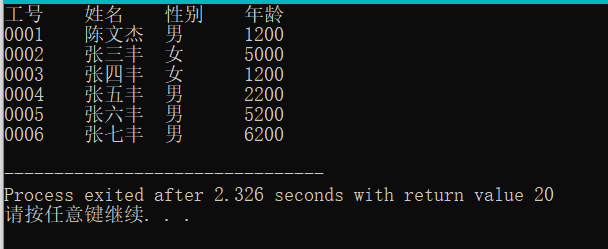#### 结构体的应用

##### 案例一、

1、编写一个函数，它的目的是为了输出所有性别为男的的职工信息
算法分析：
1)这个函数它不用返回值因为该函数是为了完成输出
2）该函数要处理很多职工，就需要一个数组，数组的类型为职工类型
注意：在C语言当中字符串不能直接比较是否相同，要通过strcmp这个函数来比较其两个字符串是否相同

#include<stdio.h>
#include<string.h>
typedef struct worker{
char id;
char name;
char sex;
int pay;
}WOR;
void QueryWorker(WOR a[],int n){//次函数是用来输出长度为n的工人数组的信息
int i;
printf("全部员工：\n");
printf("工号\t姓名\t性别\t年龄\n");
for(i=0;i<6;i++){
printf("%s\t%s\t%s\t%d\n",a[i].id,a[i].name,a[i].sex,a[i].pay);
}
printf("所有男员工：\n");
printf("工号\t姓名\t性别\t年龄\n");
for(i=0;i<6;i++){
if(strcmp(a[i].sex,"男")==0){
printf("%s\t%s\t%s\t%d\n",a[i].id,a[i].name,a[i].sex,a[i].pay);
}
}
printf("所有女员工：\n");
printf("工号\t姓名\t性别\t年龄\n");
for(i=0;i<6;i++){
if(strcmp(a[i].sex,"女")==0){
printf("%s\t%s\t%s\t%d\n",a[i].id,a[i].name,a[i].sex,a[i].pay);
}
}
}
void main(){
WOR a={
{"0001","陈文杰","男",1200},
{"0002","张三丰","女",5000},
{"0003","张四丰","女",1200},
{"0004","张五丰","男",2200},
{"0005","张六丰","男",5200},
{"0006","张七丰","男",6200}
};
QueryWorker(a,6);
}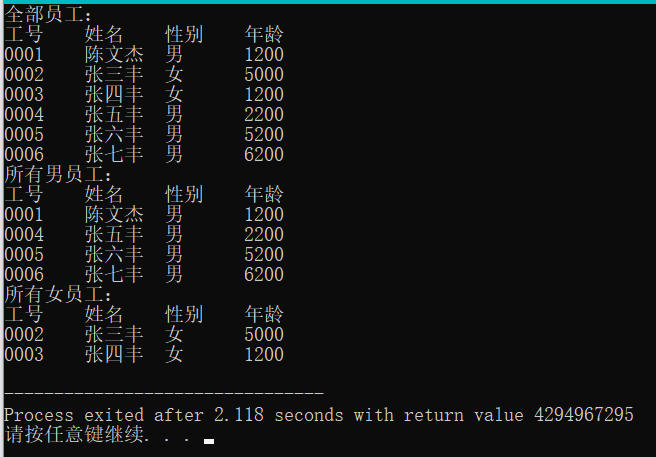2、能够输出指定性别的职工

#include<stdio.h>
typedef struct worker{
char id;
char name;
char sex;
int pay;
}WOR;
void QueryBySex(WOR a[],int n,char b[]){//次函数是用来输出长度为n的工人数组的信息
int i;

printf("工号\t姓名\t性别\t年龄\n");
for(i=0;i<6;i++){
if(strcmp(a[i].sex,b)==0){
printf("%s\t%s\t%s\t%d\n",a[i].id,a[i].name,a[i].sex,a[i].pay);
}
}

}
void main(){
WOR a={
{"0001","陈文杰","男",1200},
{"0002","张三丰","女",5000},
{"0003","张四丰","女",1200},
{"0004","张五丰","男",2200},
{"0005","张六丰","男",5200},
{"0006","张七丰","男",6200}
};
char b;
printf("请输入您要查询的性别 :\n");
scanf("%s",b);
QueryBySex(a,6,b);
}


#### 案例二、

让用户输入一个工号，然后在一个结构体数组当中去查找有没有这个工人，如果有则输出这个工人的信息，如果没有则找不到此人

#include<stdio.h>
typedef struct worker{
char id;
char name;
char sex;
int pay;
}WOR;

void FindById(WOR a[],int n,char id[]){
//我们要访问数组里面每一个工人，如果发现了一个工人，就退出循环。如果找不到就输出找不到此人
int i;
for(i=0;i<n;i++){
if(strcmp(a[i].id,id)==0){//说明第i个工人就是我们要找的职工
break;//强制终止循环
}
}

//在退出循环之后，再来检查i与n的关系
if(i<n){//说明循环在中途就break,发现了职工
printf("%s\t%s\t%s\t%d\n",a[i].id,a[i].name,a[i].sex,a[i].pay);
}
else//找不到职工
{
printf("找不到此人");
}
}

void main(){
WOR a={
{"0001","陈文杰","男",1200},
{"0002","张三丰","女",5000},
{"0003","张四丰","女",1200},
{"0004","张五丰","男",2200},
{"0005","张六丰","男",5200},
{"0006","张七丰","男",6200}
};
char b;
printf("请输入您要查询的id :\n");
gets(b);
FindById(a,6,b);
}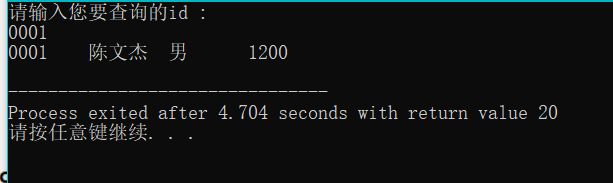#### 案例三、

求出所有职工中最高工资那个职工的信息
注意：这里有一个知识点：成员比较整体赋值

#include<stdio.h>
#include<string.h>
typedef struct worker{
char id;
char name;
char sex;
int pay;
}WOR;
void max(WOR a[],int n){
WOR m=a;
int i;
for(i=0;i<n;i++){
if(m.pay<a[i].pay){//如果m的工资小于a[i]的工资，就把m赋值给a[i]
m=a[i];
}
}
printf("%s\t%s\t%s\t%d\n",m.id,m.name,m.sex,m.pay);
//假设第一个工人是最高工资的那个工人
}
void main(){
WOR a={
{"0001","陈文杰","男",1200},
{"0002","张三丰","女",7000},
{"0003","张四丰","女",1200},
{"0004","张五丰","男",2200},
{"0005","张六丰","男",5200},
{"0006","张七丰","男",6200}
};
max(a,6);
}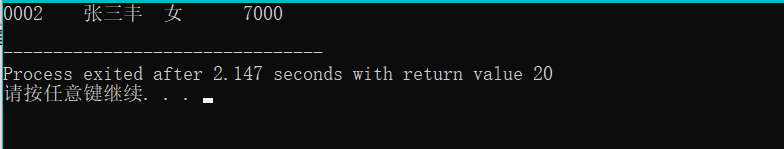上方程序改进
输出所有工资大于平均工资的职工
1、必须要先求出平均
2、在访问每一个职工，如果发现这个职工的工资要小于平均工资的话，就输出这个职工的信息。

#include<stdio.h>
#include<string.h>
typedef struct worker{
char id;
char name;
char sex;
int pay;
}WOR;
void QueryByAver(WOR a[],int n){
int i;
float aver;
int sum = 0;
//求出总工资
for(i=0;i<n;i++){
sum+=a[i].pay;
}
//求出平均工资aver
aver =(float)sum/n;
printf("平均工资为%f\n",aver);
printf("大于平均工资为：\n");
//访问每一个职工
for(i=0;i<n;i++){
if(a[i].pay>=aver){//如果发现职工的工资大于等于平局工资，就输出对应职工的信息
printf("%s\t%s\t%s\t%d\n",a[i].id,a[i].name,a[i].sex,a[i].pay);
}
}
}
void main(){
WOR a={
{"0001","陈文杰","男",3200},
{"0002","张三丰","女",7000},
{"0003","张四丰","女",1200},
{"0004","张五丰","男",2200},
{"0005","张六丰","男",5200},
{"0006","张七丰","男",6200}
};
QueryByAver(a,6);
}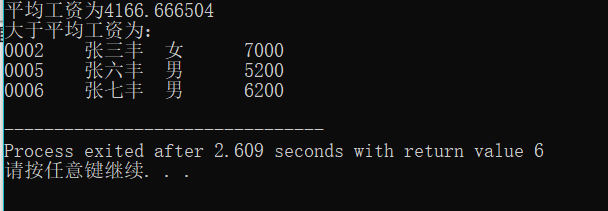算法变换

#include<stdio.h>
#include<string.h>
typedef struct stud{//定义了一个结构体
char Id;//学号
char Name;//姓名
int China;//语文成绩
int Math;//数学成绩
int English;//英语成绩
}STU;
//编写一个函数，它的目的是为了输出所有学生的总分与平均分
void fun(STU a[],int n){
int sum=0,i;
printf("学号\t姓名\t语文\t数学\t英语\t总分\t平局分\n");
//要访问里面的每一个学生
for(i=0;i<n;i++){
//对于一个学生a[i]而言我们要来输出它的信息
printf("%s\t%s\t%d\t%d\t%d\t%d\t%.1f\n",
a[i].Id,a[i].Name,a[i].China,a[i].Math,a[i].English,
a[i].China+a[i].Math+a[i].English,
(a[i].China+a[i].Math+a[i].English)/3.0);
sum = a[i].China+a[i].Math+a[i].English;
}
}
void main(){
STU a={
{"S1","张一军",127,150,100},
{"S2","张二军",75,85,89},
{"S3","张三军",67,58,86},
{"S4","张四军",120,101,87},
{"S5","张五军",136,100,82},
{"S6","张六军",141,52,83},
{"S7","张七军",125,85,81},
{"S8","张八军",136,65,79}
};
fun(a,8);
}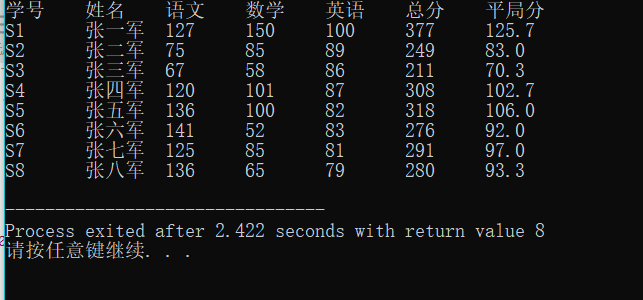案例变换：
注意字符串也不能够直接赋值，要通过一个函数来对字符串进行赋值
strcpy:通过这个函数才可以给字符串进行赋值

#include<stdio.h>
#include<string.h>
typedef struct stud{//定义了一个结构体
char Id;//学号
char Name;//姓名
int China;//语文成绩
int Math;//数学成绩
int English;//英语成绩
}STU;
//编写一个函数，求出每一个学生的优秀科目，目的不是输出分数，而是要输出对应科目的名称
void Perferct(STU a[],int n){
int sum=0,i;
char per;
int maxscore;//用来确保最优科目的分数
//要输出标题
printf("学号\t姓名\t语文\t数学\t英语\t最优秀科目\n");
//要访问里面的每一个学生，对于第i学生要确认它的最优科目名称（语文，数学，英语）
//科目名称是字符串，所以要先定义一个字符串来确保保存最优科目名称
for(i=0;i<n;i++){
maxscore=a[i].China;//认为第i个学生的语文是最优秀的
strcpy(per,"语文");
if(maxscore<a[i].Math){
maxscore = a[i].Math;
strcpy(per,"数学");
}
if(maxscore<a[i].English){
maxscore = a[i].Math;
strcpy(per,"英语");
}
printf("%s\t%s\t%d\t%d\t%d\t%s\n",
a[i].Id,a[i].Name,a[i].China,a[i].Math,a[i].English,per);
}
}

void main(){
STU a={
{"S1","张一军",127,150,100},
{"S2","张二军",75,85,89},
{"S3","张三军",67,58,86},
{"S4","张四军",120,101,87},
{"S5","张五军",136,100,82},
{"S6","张六军",141,52,83},
{"S7","张七军",125,85,81},
{"S8","张八军",136,65,79}
};
Perferct(a,8);
}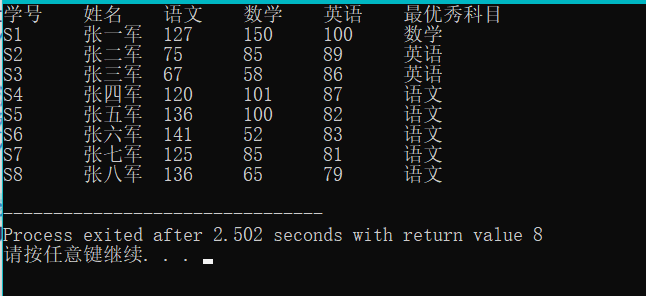展开全文c语言 程序设计 指针 编程语言
• 为了定义结构，必须使用 struct 语句。struct 语句定义了一个包含多个成员的新的数据类型，struct 语句的格式如下： struct type_name { member_type1 member_name1; member_type2 member_name2; member_type3 ...
• ## 结构体及结构体数组的定义

万次阅读 多人点赞 2020-07-19 23:29:15
1.结构体 结构体是用户自定义的可用的数据类型，它允许您存储不同类型的数据项。...定义结构体变量: struct STU stu1,stu2; （2）定义了一个结构体STU的同时定义了两个结构体STU的变量stu2，stu3。 struct结构体 struct
• #include <stdio.h> #include <...// 定义结构体 typedef struct{ char *name; int age; }Student; // 结构体数组输入 void inputStructArray(Student students[],int size){ int i=0; ...数据结构
•c语言
• 一、结构体 数组 作为函数参数 ( 数组 在 栈内存创建 )、 二、完整代码示例结构体 结构体别名
• 如果要处理多个类型相同的结构体数据，就需要定义结构体数组 两种结构体数组的定义形式 结构体数组定义的方法和结构体变量定义的方法一样，只不过加了一个数组的长度而已 第一种：和结构体类型同时定义，例如： ...c语言 开发语言 算法
• C语言之结构体数组定义一个存储5个员工信息（包含编号，姓名，工资）的结构体数组c语言 开发语言 后端
• 1. 什么场合会用到结构体数组 举个例子，我控制13个...2.1先定义结构体类型，后定义结构体数组 struct UploadFormat { uint8 head; uint16 eco2; uint8 checksum; }; struct UploadFormat line; 2.2在...c++ c语言
• 与一般指针类似结构体也可以使用结构体指针进行引用使用结构体指针的定义方式如下： struct Student stu = { 1001,"Li Lei",'M',1.87 }; //定义一个Student 的结构体变量stu struct Student *p=&stu; //...
• 结构体变量以及结构体数组 1、概述 C 语言允许用户自己指定这样一种数据结构，它由不同类型的数据组合成一个整体，以便引用，这些组合在一个整体中的数据是互相联系的，这样的数据结构称为结构体，它相当于其它高级...c语言 单片机
• ## 结构体数组初始化

千次阅读 2021-03-17 15:24:52
《代码大全》建议在变量定义的时候进行初始化，但是很多人，特别是新人对结构体或者结构体数组定义是一般不会初始化，或者不知道怎么初始化。 1、初始化 typedef struct _TEST_T { int i; char c; }TEST_T; TEST...数据结构 编程语言
• 使用typedef的情况二、结构体数组三、结构体嵌套结构体 一、结构体的定义使用 结构体是存储不同类型的数据项 结构体是一种构造数据类型 结构体中的成员空间是独立的 为了访问结构的成员，我们使用成员...c语言
• 一、下标手动给字符数组赋值>二、通过strcpy给赋值>三、结构体数组赋值>四、作业c++ 反汇编 堆栈 内存结构
• #include <stdio.h> #include <stdlib.h> typedef struct sf { int n; char c;... //定义结构体sf，给他起个别名fc int main ( int argc, char *argv[] ) { int i...
• C语言sizeof求结构体数组长度C语言sizeof求结构体数组长度#include typedef struct ss{char a;//偏移量为0，大小为1,0能被1整除，=1short b;//偏移量为1，大小为2,1不能被2整除，=1+1+2=4int c;//偏移量为4，大小为4...
• C语言实现动态结构体数组原因是因为以前使用C++来实现的，主要VERCTOR C++标准库的失代器而不是纯C；C++是C语言的超集，除了包含C外，还有C++各种新语法，新库！不过虽然C++起来简单，开发效率高，不过相对C语言...
• 示例代码： ...typedef void (*hanshuzhizhen) (void); struct jiegouti//这是个结构体 { int8_t paraone;//第一个参数 uint8_t paratwo;//第二个参数 uint16_t parathree;//第三个参数 hansh...C语言
• ## 结构体和结构体数组

千次阅读 多人点赞 2020-03-14 11:14:21
声明结构体变量时不分配空间，在定义结构体变量时才分配内存空间。 例1 学校体育考试，Tom得了一个不错的分数，那么请一个结构体类型保存并输出到控制台 提示：声明student是一个结构体类型；存储如上图一名...
• 1、结构体数组的妙可以巧妙的实现一个功能模块的调用 1）、将需要关联的变量和数据放到一个结构体里面。 2）、通过给结构体添加一个枚举实现对于结构体里面其他数据的快速遍历。 3）、结合switch的形式来实现对于...
• typedef struct _TEST_T { int i; char c; }TEST_T; TEST_T gst = {.i = 1, .c = "12345"}; TEST_T gst = {1, “12345”};//可以初始化，设置i为1，s为一个字符串. TEST_T gst = {1};//初始化个数少于实际...开发语言 后端
• typedef struct student//定义结构体 { char stu_number; char stu_name; float usual_mark; float exam_mark; float overall_mark; }Student; //创建动态结构体数组 stus=(Student*)malloc(sizeof...c语言
• 你好，请教一下： typedef struct {ElemType data; int cur; }compoent,SLinkList{MAXSIZE}; 代码的作用是什么？c++...